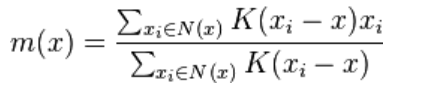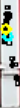# 【算法实现】Meanshift 求2d散点的密度最大处，点最密集处

【Python Meanshif】

1、meanshift 目前有几个比较常用的接口提供： Scikit-Learn，Opencv

2、meanshift 在追踪领域，聚类等都有着比较多的应用

3、本次主要介绍meanshift的算法逻辑，以及以及简单的实现方法：

1）输入一堆2D点集记为X

2）设置初始点坐标，建议选择，X的均值点记为x_mean，同时设置一个距离阈值，选择高斯函数作为权重核函数

3）输入其实点位置iter_position,根据距离阈值求出所有近邻点N(X)，然后根据如下公式求出这些点的带权中心4）用求得的m(x)来更新iter_position，然后重复3) ，根据迭代次数限制，或者判断 m(x)与iter_position 距离变动大小来决定推出迭代，从而我们就得到了2d散点集合种，密度最大的位置，也就是点最密集的位置

下图中黑色点表示 图中 黑色点为所有数据点，蓝色点为最开始初始化的位置，黄色点为5次迭代之后找到的密度最大位置。#以下函数 主要来自于参考链接内容中的 大佬写的函数，输入数据是numpy array的2d点，shape like（30，2）
def mean_shift(data,iter_position):

look_distance = 500  # 设置近邻点搜索阈值
kernel_bandwidth = 25  # 设置权重函数（高斯）的一个阈值

def euclid_distance(x, xi):  #求 两点之间的欧式距离
return np.sqrt(np.sum((x - xi)**2))

def neighbourhood_points(X, x_centroid, distance = 5): #求N（X）近邻点集合
eligible_X = []
for x in X:
distance_between = euclid_distance(x, x_centroid)
if distance_between <= distance:
eligible_X.append(x)
return eligible_X

def gaussian_kernel(distance, bandwidth): #权重函数，对应到公式中的k（x-xi）
val = (1/(bandwidth*math.sqrt(2*math.pi))) * np.exp(-0.5*((distance / bandwidth))**2)
return val

X = np.copy(data)
n_iterations = 5  #迭代次数
for it in range(n_iterations):
neighbours = neighbourhood_points(X, iter_position, look_distance)
#print(neighbours)
numerator = 0.
denominator = 0.
for neighbour in neighbours:
distance = euclid_distance(neighbour, iter_position)
weight = gaussian_kernel(distance, kernel_bandwidth)
numerator += (weight * neighbour)
denominator += weight
new_x = numerator / denominator
iter_position=new_x

return iter_position